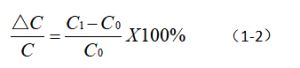Characterization parameters of electrolytic capacitors-Leakage Current, Rated Voltage, Capacitance Temperature Change Rate.

🔔 Today we will introduce: leakage current (I), rated voltage (U), and capacitance temperature change rate.

🔺1.1.1 Leakage current (I)

📌The leakage current of an electrolytic capacitor is used to characterize its insulation, which is equivalent to the insulation resistance parameter of other types of capacitors. The leakage current value of an electrolytic capacitor is closely related to its capacitance and applied voltage (not greater than the rated voltage), so the allowable maximum value should be lower than the calculated value of formula (1-1)🔺1.1.2 Rated voltage (U)

📌The rated voltage of an electrolytic capacitor is the highest DC voltage that can be continuously applied to the capacitor at the rated temperature. Although the rated voltage is not a parameter to measure the limit characteristics of a capacitor, it is generally stipulated that the working voltage value applied to the capacitor should be lower than the rated voltage value. Reducing the applied voltage has a significant effect on improving the service life of the product.

🔺1.1.3 Temperature change rate of capacitance

📌The capacitance of the capacitor will change accordingly with the change of temperature. Generally, the percentage of capacitance temperature change (1-2) is used.Among them: C0 is the capacitance at temperature, and C1 is the capacitance at the positive or negative limit temperature.

🔺In addition, the operating temperature range and maximum ripple current of the electrolytic capacitor are also important parameters. Generally, the operating temperature range of ordinary electrolytic capacitors is -40~+85℃, and the operating temperature range of special purpose capacitors has different requirements. For example, the upper limit operating temperature of high-temperature electrolytic capacitors is +105℃ or +125℃, and that of wide-temperature electrolytic capacitors The operating temperature range is -55~+125℃. The higher the upper limit working temperature of the electrolytic capacitor, the more it is necessary to increase the withstand voltage of the anodic oxide film when the capacitor is designed, so that the capacitor can work at a higher ambient temperature and has a longer working life. The maximum ripple current refers to the maximum value of the AC current allowed by the capacitor, which is closely related to the equivalent series resistance of the electrolytic capacitor. The lower the equivalent series resistance value of the electrolytic capacitor, the higher the ripple current value allowed to pass.

🔺Summary: The characteristic parameters of electrolytic capacitors are: Stadand Capacitance and allowable Tolerance, Loss Tangent Value, Leakage Current, Rated Voltage, Capacitance Temperature Change Rate, Operating Temperature and Ripple Current.